• Java-求根号n(示例代码)

千次阅读 2021-02-26 13:57:00
平方，开根号java中是很简单的，Math.sqrt(double n)或者 Math.pow(double a, double b)，a的b次方。但是我们可以自己想想，这些方法到底是怎么实现的。就拿开根号来解释，它有两种方法，二分法和牛顿迭代法。...

平方，开根号在java中是很简单的，Math.sqrt(double n)或者 Math.pow(double a, double b)，求a的b次方。但是我们可以自己想想，这些方法到底是怎么实现的。

就拿开根号来解释，它有两种方法，二分法和牛顿迭代法。

二分法：

比如求根号5

第一步:折半：       5/2=2.5

第二步:平方校验:  2.5*2.5=6.25>5，并且得到当前上限2.5，记录。

第三步:再次向下折半:2.5/2=1.25

第四步:平方校验：1.25*1.25=1.5625<5,得到当前下限1.25，记录

第五步:再次折半:2.5-(2.5-1.25)/2=1.875

第六步:平方校验：1.875*1.875=3.515625<5,得到当前下限1.875，替换下限值

......

一直到与5的差值在你定义的误差范围内才结束循环

代码：

importjava.text.DecimalFormat;public classMain {public static double sqrt(doublenum){if(num<0) {return -1;

}double low = 0;double high = num/2;double precision = 0.000001;

//格式化，保证输出位数

DecimalFormat df= new DecimalFormat("#.00");double res =high;while(Math.abs(num-(res*res))>precision) {if(high*high >num) {double n= high - (high-low)/2;if(n*n>num) {

high=n;

}else if(n*n

low=n;

}else{returnDouble.valueOf(df.format(n));

}

res=n;

}else if(high*high num) {

low=high;

high=m;

}else if(m*m

low=high;

high=m;

}else{returnDouble.valueOf(df.format(m));

}

res=m;

}else{returnDouble.valueOf(df.format(high));

}

}returnDouble.valueOf(df.format(res));

}public static voidmain(String[] args) {double a = 7;

System.out.println(sqrt(37));

}

}

牛顿迭代法：

其实就是逼近的思想，例如我们要求a的平方根，首先令f(x)=x^2-a，那么我们的目的就是求得x使得f(x)=0，也就是求x^2-a这条曲线与x轴的交点，画图举例：由函数f(x)=x^2-a，我们求导可以知道，函数上任意一点(x,y)的切线的斜率为2x。假设过点(x0,y0)的切线方程为y=kx+b，那么切线与x轴的交点横坐标为-b/k。而b=y0-kx0,k=2x0,y0=x0^2-a,化简-b/k=(x0+a/x0)/2。

也就是说(x0+a/x0)/2是过点(x0,y0)的切线与x轴的交点的横坐标。记(x0+a/x0)/2=x‘,继续求过点(x‘,f(x‘))的切线与x轴的交点的横坐标x‘‘，很明显x‘‘比x‘更靠近函数f(x)=x^2-a与x轴的交点的横坐标(即a的正平方根)。逐渐的逼近f(x)=0;

所以公式为：x‘ = (x‘+a/x‘)/2。

代码：

importjava.text.DecimalFormat;public classMain1 {public static double sqrt(doublex) {if(x<0) {return -1;

}//格式化，保证输出位数

DecimalFormat df = new DecimalFormat("#.00");double k =x;double precision = 0.000001;while((k*k-x)>precision) {

k=0.5*(k+x/k);

}returnDouble.valueOf(df.format(k));

}public static voidmain(String[] args) {double a = 9;

System.out.println(sqrt(a));

}

}

参考文献：

展开全文java求根号值
• java如何开根号？

千次阅读 2020-12-21 23:00:53
展开全部JAVA凡是涉及数学的符号前面都要加MATH。class A{public static void main(){double m=4.0;double n=Math.sqrt(m);System.out.println(n);}}扩展资料：java实现开根62616964757a686964616fe59b9ee7ad...

展开全部

JAVA凡是涉及数学的符号前面都要加MATH。

class A{

public static void main(){

double m=4.0;

double n=Math.sqrt(m);

System.out.println(n);

}

}

扩展资料：

public static void main(String[] args) { long start = System.currentTimeMillis(); double

target=9876543212345d; double result =sqrt(target);

System.out.println("sqrt耗时："+(System.currentTimeMillis()-start)+",result:"+result);

start=System.currentTimeMillis();

result =SqrtByBisection(target, 0);

System.out.println("SqrtByBisection耗时："+(System.currentTimeMillis()

start)+",result:"+result);

start=System.currentTimeMillis();

result = SqrtByNewton(target, 0);

System.out.println("SqrtByNewton耗时："+(System.currentTimeMillis()

start)+",result:"+result);

}

展开全文java 开根号函数
• java实现开根号的运算

万次阅读 2020-04-21 22:43:37
java实现开根号的运算 如果某个数字正好可以开根号为2个整数，例如1,4,9等，那就很简单了。 如果某个数字不可以正好开根号为2个整数，而且要保留几位精度，例如：2,3,5等，我们该怎么办呢？？？？？ 首先我们可以...

今天我们来看一下这道题，java如何实现开根号的运算。大家都知道，java,c++,python等都有直接的函数可以给我们用，但是面试官这个时候让我们手撕源码，这个时候怎么做呢，来，不要慌！！！如果某个数字正好可以开根号为2个整数，例如1,4,9等，那就很简单了。

如果某个数字不可以正好开根号为2个整数，而且要保留几位精度，例如：2,3,5等，我们该怎么办呢？？？？？

首先我们可以把这个数字分成整数部分和小数部分，分别计算。

例如√5≈2.236  我们可以先算出整数部分为2，然后在根据保留几位精度，去计算小数部分。依次计算十分位、百分位和千分位等，然后把整数位+十分位+百分位+千分位+。。。，结果就是我们想要的结果了。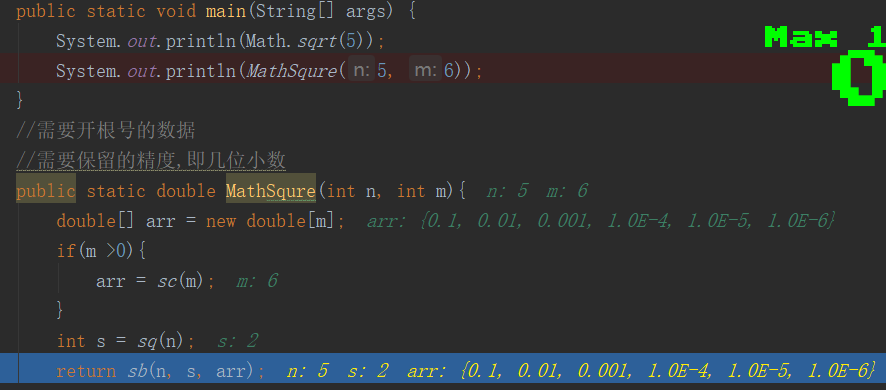来看代码

import java.math.BigDecimal;

/**
* @Auther: liuhaidong
* Data: 2020/4/21 0021、20:55
* Description:
* @version: 1.0
*/
public class Test6 {
public static void main(String[] args) {
System.out.println(Math.sqrt(5));
System.out.println(MathSqure(5, 6));
}
//需要开根号的数据
//需要保留的精度,即几位小数
public static double MathSqure(int n, int m){
double[] arr = new double[m];
if(m >0){
arr = sc(m);
}
int s = sq(n);
return sb(n, s, arr);
}
/**
* 计算要保留几位小数
* @param m
* @return
*/
public static double[] sc(int m){
double[] arr = new double[m];
int num = 0;
while(num != m){
double f = 1;
for(int i=0;i<=num;i++){
f = f*10;
}
arr[num] = 1/f;
num++;
}
return arr;
}
/**
* 计算整数位
* @param n
* @return
*/
public static int sq(int n){
if( n == 1){
return 1;
}
int tmp = 0;
for(int i=1;i<=n/2+1;i++){
if(i*i == n){
tmp = i;
break;
}
if(i*i > n){
tmp = i-1;
break;
}
}
return tmp;
}

/**
* 开根号
* @param n
* @param j
* @param arr
* @return
*/
public static double sb(int n, double j, double[] arr){
double tmp = j;
for(int p=0;p<arr.length;p++){
if(p>0){
j = tmp;//计算过后的值（整数位+小数位的和，赋值给j，下面继续运算）
}
for(int i=1;i<=9;i++){//小数位只有九位{0.1,0.2,0.3,0.4,0.5,0.6,0.7,0.8,0.9}
tmp = i*arr[p]+j;//i*arr[p],相当于每次加0.1,0.2 ...
if(tmp*tmp == n){
return tmp;
}
if(tmp*tmp >n){
//避免丢失精度
BigDecimal c1 = new BigDecimal(Double.toString(tmp));
BigDecimal c2 = new BigDecimal(Double.toString(arr[p]));
tmp = c1.subtract(c2).doubleValue();
break;
}
}
}
return tmp;
}
}

思路继续讲解。

小于2.3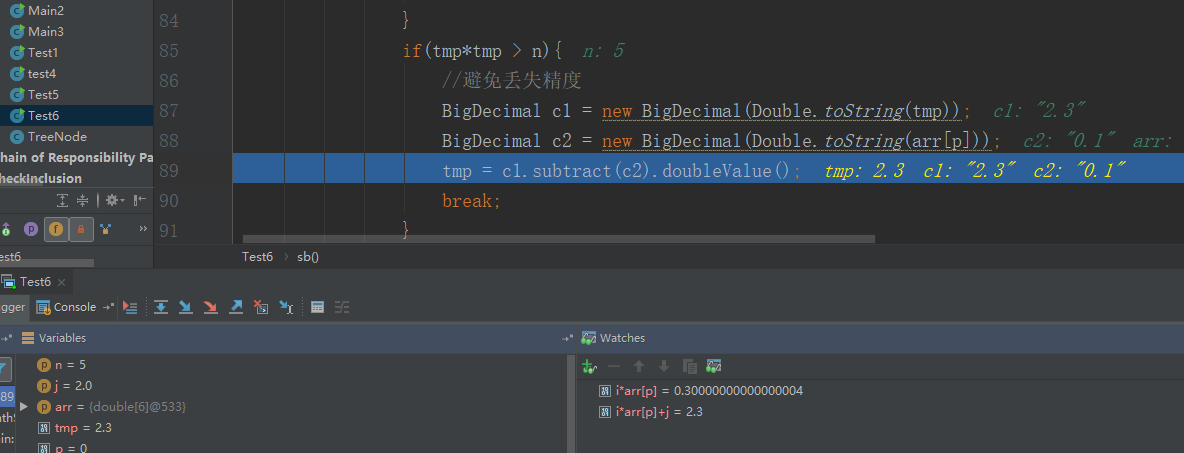把2.2记录在这里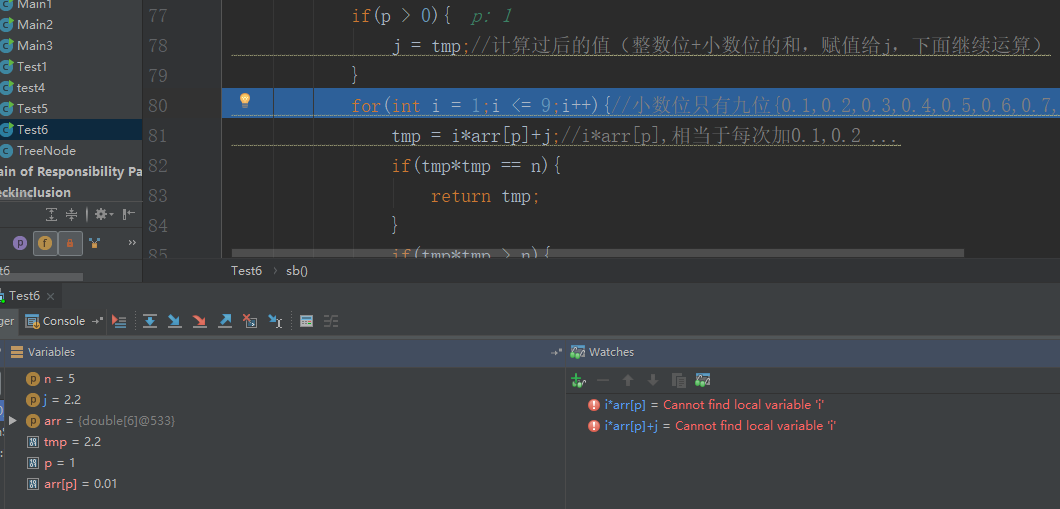小于2.24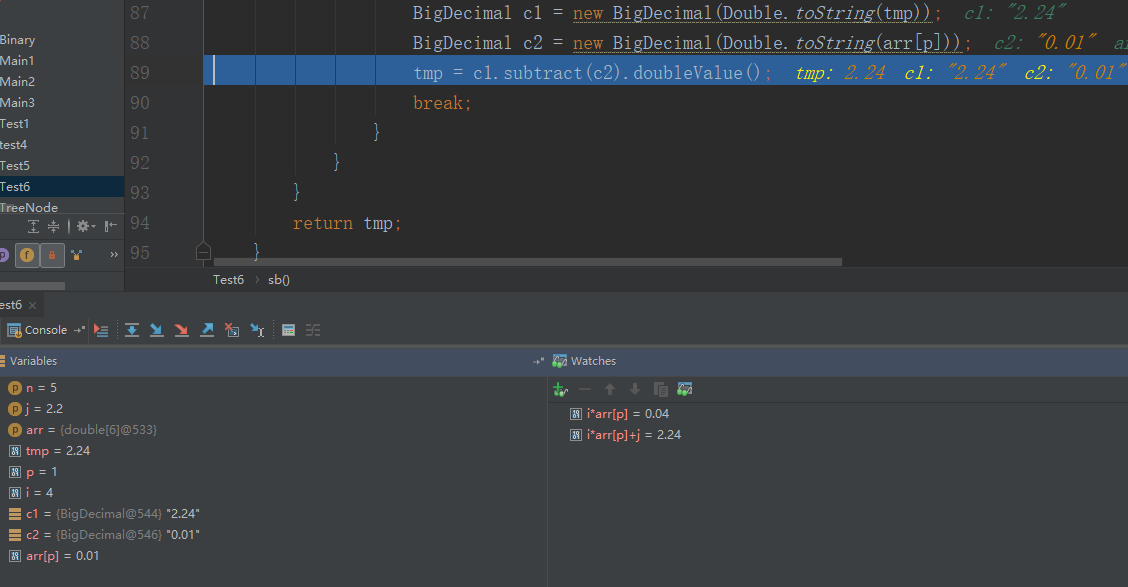记录2.23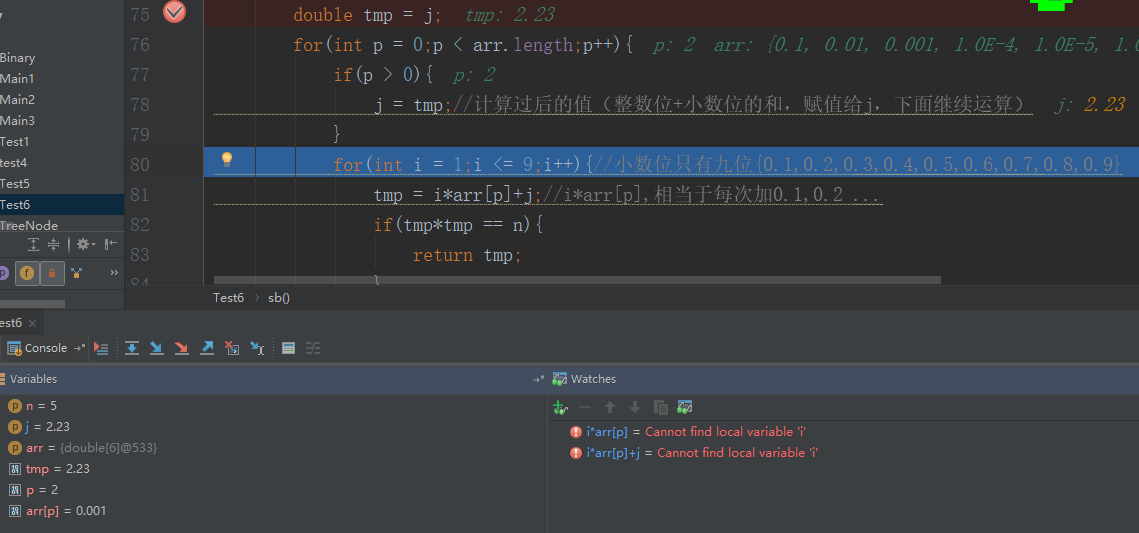依次这样做。本公众号分享自己从程序员小白到经历春招秋招斩获10几个offer的面试笔试经验，其中包括【Java】、【操作系统】、【计算机网络】、【设计模式】、【数据结构与算法】、【大厂面经】、【数据库】期待你加入！！！

展开全文开根号
• Java-求根号n

2020-12-23 22:20:04
平方，开根号java中是很简单的，Math.sqrt(double n)或者 Math.pow(double a, double b)，a的b次方。但是我们可以自己想想，这些方法到底是怎么实现的。就拿开根号来解释，它有两种方法，二分法和牛顿迭代法。...

平方，开根号在java中是很简单的，Math.sqrt(double n)或者 Math.pow(double a, double b)，求a的b次方。但是我们可以自己想想，这些方法到底是怎么实现的。

就拿开根号来解释，它有两种方法，二分法和牛顿迭代法。

二分法：

比如求根号5

第一步:折半：       5/2=2.5

第二步:平方校验:  2.5*2.5=6.25>5，并且得到当前上限2.5，记录。

第三步:再次向下折半:2.5/2=1.25

第四步:平方校验：1.25*1.25=1.5625<5,得到当前下限1.25，记录

第五步:再次折半:2.5-(2.5-1.25)/2=1.875

第六步:平方校验：1.875*1.875=3.515625<5,得到当前下限1.875，替换下限值

......

一直到与5的差值在你定义的误差范围内才结束循环

代码：

importjava.text.DecimalFormat;public classMain {public static double sqrt(doublenum){if(num<0) {return -1;

}double low = 0;double high = num/2;double precision = 0.000001;

//格式化，保证输出位数

DecimalFormat df= new DecimalFormat("#.00");double res =high;while(Math.abs(num-(res*res))>precision) {if(high*high >num) {double n= high - (high-low)/2;if(n*n>num) {

high=n;

}else if(n*n

low=n;

}else{returnDouble.valueOf(df.format(n));

}

res=n;

}else if(high*high num) {

low=high;

high=m;

}else if(m*m

low=high;

high=m;

}else{returnDouble.valueOf(df.format(m));

}

res=m;

}else{returnDouble.valueOf(df.format(high));

}

}returnDouble.valueOf(df.format(res));

}public static voidmain(String[] args) {double a = 7;

System.out.println(sqrt(37));

}

}

牛顿迭代法：

其实就是逼近的思想，例如我们要求a的平方根，首先令f(x)=x^2-a，那么我们的目的就是求得x使得f(x)=0，也就是求x^2-a这条曲线与x轴的交点，画图举例：由函数f(x)=x^2-a，我们求导可以知道，函数上任意一点(x,y)的切线的斜率为2x。假设过点(x0,y0)的切线方程为y=kx+b，那么切线与x轴的交点横坐标为-b/k。而b=y0-kx0,k=2x0,y0=x0^2-a,化简-b/k=(x0+a/x0)/2。

也就是说(x0+a/x0)/2是过点(x0,y0)的切线与x轴的交点的横坐标。记(x0+a/x0)/2=x',继续求过点(x',f(x'))的切线与x轴的交点的横坐标x''，很明显x''比x'更靠近函数f(x)=x^2-a与x轴的交点的横坐标(即a的正平方根)。逐渐的逼近f(x)=0;

所以公式为：x' = (x'+a/x')/2。

代码：

importjava.text.DecimalFormat;public classMain1 {public static double sqrt(doublex) {if(x<0) {return -1;

}//格式化，保证输出位数

DecimalFormat df = new DecimalFormat("#.00");double k =x;double precision = 0.000001;while((k*k-x)>precision) {

k=0.5*(k+x/k);

}returnDouble.valueOf(df.format(k));

}public static voidmain(String[] args) {double a = 9;

System.out.println(sqrt(a));

}

}

参考文献：

展开全文java 根号x
• 匿名用户1级2014-05-25 回答在java.lang包中有个public final Math类，类中函数如下static double abs(double a)返回 double 值的绝对值。static float abs(float a)返回 float 值的绝对值。static int abs(int a)...java 开根号函数
• java 不用内置函数开根号

千次阅读 2016-08-20 16:47:22
如题，刚开始用循环直接根号，后来模拟了内置函数sqrt取 主要使用二分法，均已经运行可以出结果 直接上代码： 第一个： package test; import java.util.Scanner; public class xy { public static ...java 算法 二分法
• 最受欢迎的Java面试问题之一是，“给定一个整数x，编写一个Java程序来找到它的平方根”。解决这个问题有很多方法。在本文中，让我们看看在Java中查找平方根和平方根的不同方法。最受欢迎的Java面试问题之一是，...java开根号函数
• 使用Java自己实现开根号运算，网上也有不少代码，多数都使用String或者数组。这里写一段只使用double基础数据类型实现的方法。private static double sqrt(int n, int p) {double lower = 0;while (lower * lower &...java 开根号
• package classifier; public class getSqrt { public static void main(String[] args){ double a = 15; System.out.println(Math.sqrt(a)); System.out.println(Math.floor(Math.sqr...
• 【java】分段函数求值

千次阅读 2020-02-20 11:54:39
分段函数求值 题目描述 有一个函数 y={ x , x<1 | 2x-1 ， 1<=x<10 \ 3x-11 ， x>=10 写一段程序，输入x，输出y import java.util.Scanner; public class Main { public static void main(String []...
• java里实现开根号

千次阅读 2021-02-26 10:26:28
java里实现开根号[2021-02-03 08:57:13]简介:php去除nbsp的方法：首先创建一个PHP代码示例文件；然后通过“preg_replace("/(\s|\&nbsp\;|　|\xc2\xa0)/", " ", strip_tags(\$val));”方法去除所有nbsp即可。推荐...java 开三次根号
• java实现开根号

万次阅读 2017-08-22 20:46:51算法 java
• import java.util.LinkedList; import java.util.List; public class Calc { final public static String[] OPT_ADD = { "+", "add", "plus", "jia" }; final public static String[] OPT_SUB = { "-", "sub", ...
• Java实现开根号

千次阅读 2018-09-30 02:21:47
Java实现开根号 二分法 static float sqr(float x){ float max = x; float min = 0; float mid=(min+max)/2; while (true){ if (Math.abs(x-mid*mid)&amp;lt;0.002) return mid;...开根号
• 原标题：面试题：如何求根号2Great Eagle 程序猿DD来源：算法面试题问题小E最近找实习的时候，被面试官问了这样一道题：如何求根号2的值？小E没能答上来，回来后向老师请教。思路 点评：以上介绍了二分法和牛顿迭代...
• 数学运算的指数函数方法相关说明源码运行结果 相关说明 方法Math.A A为各种数学方法 数学方法(这里的A）： exp(a) ：e的a次方 log(b) ：ln(b)的值 lgc ：lgc的值 sqrt(d) ：根号d的结果，且d不为负数 cbrt(h)...java 数学运算
• 设f(x)=x 3-y, f(x)=0时的解x，即为y的立方根。 根据牛顿迭代思想，x n+1=x n-f(x n)/f'(x n)即x=x-(x 3-y)/(3*x 2)=(2*x+y/x/x)/3; import java.util.*; public class Main{ public static void main(String[] ...
• java中sqrt函数的详解

万次阅读 2017-08-14 18:41:06
首先要明白，牛顿迭代法的是函数和X轴的交点的横坐标，也就是我们说的根 1）那么第一步就是构建曲线了。 假设有一个数c，我们它的平方根x，那么有一个等式，x^2 = c；挪到一边就是 f = x^2 - c的根x 2）...sqrt java 平方根
• 1.0的平方根是：1.02.0的平方根是：1.4142150878906253.0的平方根是：1.73204803466796884.0的平方根是：2.0000019073486335.0的平方根是：2.23606872558593756.0的平方根是：2.44948959350585947.0的平方根是：2....java开根号函数
• 逻辑比较简单, 就不作过多注释了 方法二, 牛顿迭代法 牛顿迭代法理论上是可以出绝大多数多项式的根(最低要求是该函数二阶可导, 这一点不是本文讨论的范畴, 于是略过), 大体的思想如下: 已知曲线方程f(x), 要求...
• 以上定义的方法是开根号的代码实现 public static void main(String[] args) {  System.out.println(Math.sqart(11111)); System.out.println(Mathe.squrt(11111)); System.out.println("程序没结束");...java 编程
• 小编典典安装JDK时，可以在内部找到标准库的源代码src.zip。...因此，它实际上只是一个本地调用，可能由Java在不同平台上以不同方式实现。但是，作为StrictMath状态文档：为了帮助确保Java程序的可移植...
• 例如： string = "Python is good" print(string.replace('Python','java')) 输出结果为：java is good 4、startswith 功能：用于检验在一段程序语句中，是否以特定元素（元素可以是单词、字母或数字）开头。...python根号函数
• java的math常用方法

千次阅读 2021-02-12 10:09:48
鉴于java求整时欲生欲死，整理常用math如下：1： java取整a：floor向下取整用法：Math.floor(num)Math.floor(1.9)//1 Math.floor(-1.9)//-2b: round四舍五入用法：Math.round(num)实际上是等价于Math.floor(num+0.5)...java 幂函数
• 每个语言里面基本上都有函数，不过现在好多语言里面已经慢慢的修改了它的名称，不叫函数了，而是叫做方法。为了方便起见，下面我还是以“函数”这个名称来说。我们一直在用函数，但是如果突然问你，为什么会有函数的...知识点总结
• Java求一个数的立方根

千次阅读 2019-06-09 21:20:32
求解一个数的立方根，单纯一个数学问题，我这里写两种解法。...import java.util.*; import java.text.DecimalFormat; // 格式转换，float 转为 double public class Main{ public static void mai...java 立方根...

java求根号函数java 订阅